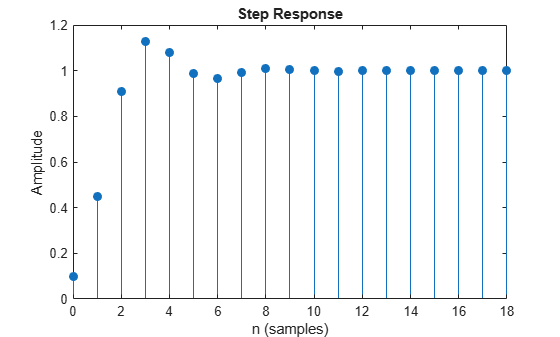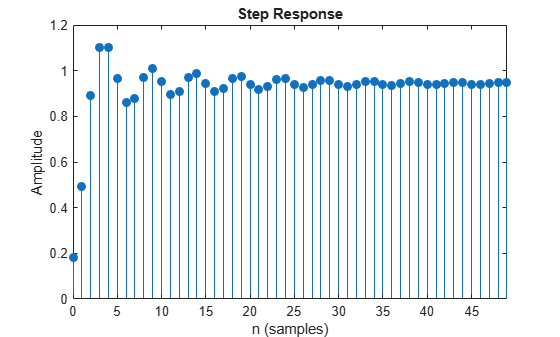# stepz

Step response of digital filter

## Syntax

``[h,t] = stepz(b,a)``
``[h,t] = stepz(sos)``
``[h,t] = stepz(d)``
``[h,t] = stepz(___,n)``
``[h,t] = stepz(___,n,fs)``
``stepz(___)``

## Description

example

````[h,t] = stepz(b,a)` returns the step response vector `h` and the corresponding sample times `t` for the digital filter with transfer function coefficients stored in `b` and `a`.```
````[h,t] = stepz(sos)` returns the step response corresponding to the second-order sections matrix `sos`.```
````[h,t] = stepz(d)` returns the step response for the digital filter `d`.```
````[h,t] = stepz(___,n)` computes the first `n` samples of the step response. This syntax can include any combination of input arguments from the previous syntaxes.```
````[h,t] = stepz(___,n,fs)` computes `n` samples and produces a vector `t` so that the samples are spaced `1/fs` units apart.```

example

````stepz(___)` with no output arguments plots the step response of the filter. If you input a `digitalFilter`, the step response is displayed in FVTool.```

## Examples

collapse all

Create a third-order Butterworth filter with normalized half-power frequency $0.4\pi$ rad/sample. Display its step response.

```[b,a] = butter(3,0.4); stepz(b,a) grid```Create an identical filter using `designfilt` and display its step response using `fvtool`.

```d = designfilt('lowpassiir','FilterOrder',3,'HalfPowerFrequency',0.4); stepz(d)```Design a fourth-order lowpass elliptic filter with normalized passband frequency $0.4\pi$ rad/sample. Specify a passband ripple of 0.5 dB and a stopband attenuation of 20 dB. Plot the first 50 samples of the filter's step response.

```[b,a] = ellip(4,0.5,20,0.4); stepz(b,a,50) grid```Create the same filter using `designfilt` and display its step response using `fvtool`.

```d = designfilt('lowpassiir','FilterOrder',4,'PassbandFrequency',0.4, ... 'PassbandRipple',0.5,'StopbandAttenuation',20, ... 'DesignMethod','ellip'); stepz(d,50)```## Input Arguments

collapse all

Transfer function coefficients, specified as vectors. Express the transfer function in terms of `b` and `a` as

`$H\left({e}^{j\omega }\right)=\frac{B\left({e}^{j\omega }\right)}{A\left({e}^{j\omega }\right)}=\frac{\text{b(1)}+\text{b(2)}\text{\hspace{0.17em}}{e}^{-j\omega }+\text{b(3)}\text{\hspace{0.17em}}{e}^{-j2\omega }+\cdots +\text{b(M)}\text{\hspace{0.17em}}{e}^{-j\left(M-1\right)\omega }}{\text{a(1)}+\text{a(2)}\text{\hspace{0.17em}}{e}^{-j\omega }+\text{a(3)}\text{\hspace{0.17em}}{e}^{-j2\omega }+\cdots +\text{a(N)}\text{\hspace{0.17em}}{e}^{-j\left(N-1\right)\omega }}.$`

Example: `b = [1 3 3 1]/6` and `a = [3 0 1 0]/3` specify a third-order Butterworth filter with normalized 3 dB frequency 0.5π rad/sample.

Data Types: `double` | `single`
Complex Number Support: Yes

Number of evaluation points, specified as a positive integer scalar or positive integer vector. If `n` is a positive integer scalar (`t` = `[0:n-1]'`), the function computes the first `n` samples of the step response. If `n` is a vector of integers, the step response is computed only at those integer values, with 0 denoting the time origin.

Data Types: `double`

Second-order section coefficients, specified as a matrix. `sos` is a K-by-6 matrix, where the number of sections, K, must be greater than or equal to 2. If the number of sections is less than 2, the function treats the input as a numerator vector. Each row of `sos` corresponds to the coefficients of a second-order (biquad) filter. The ith row of `sos` corresponds to `[bi(1) bi(2) bi(3) ai(1) ai(2) ai(3)]`.

Example: `s = [2 4 2 6 0 2;3 3 0 6 0 0]` specifies a third-order Butterworth filter with normalized 3 dB frequency 0.5π rad/sample.

Data Types: `double` | `single`
Complex Number Support: Yes

Digital filter, specified as a `digitalFilter` object. Use `designfilt` to generate a digital filter based on frequency-response specifications.

Example: ```d = designfilt('lowpassiir','FilterOrder',3,'HalfPowerFrequency',0.5)``` specifies a third-order Butterworth filter with normalized 3 dB frequency 0.5π rad/sample.

Sample rate, specified as a positive scalar. When the unit of time is seconds, `fs` is expressed in hertz.

Data Types: `double`

## Output Arguments

collapse all

Step response, returned as a column vector. If the input to `stepz` is single precision, the function computes the step response using single-precision arithmetic. The output `h` is single precision.

Sample times, returned as a vector.

## Algorithms

`stepz` filters a length `n` step sequence using

```filter(b,a,ones(1,n)) ```

and plots the results using `stem`.

To compute `n` in the auto-length case, `stepz` either uses `n = length(b)` for the FIR case, or first finds the poles using `p = roots(a)` if `length(a)` is greater than 1`.`

If the filter is unstable, `n` is chosen to be the point at which the term from the largest pole reaches 106 times its original value.

If the filter is stable, `n` is chosen to be the point at which the term due to the largest amplitude pole is 5 × 10–5 of its original amplitude.

If the filter is oscillatory (poles on the unit circle only), `stepz` computes five periods of the slowest oscillation.

If the filter has both oscillatory and damped terms, `n` is chosen to equal five periods of the slowest oscillation or the point at which the term due to the pole of largest nonunit amplitude is 5 × 10–5 times its original amplitude, whichever is greater.

`stepz` also allows for delays in the numerator polynomial. The number of delays is incorporated into the computation for the number of samples.